# An Illustrative Explanation to Dynamic Time Warping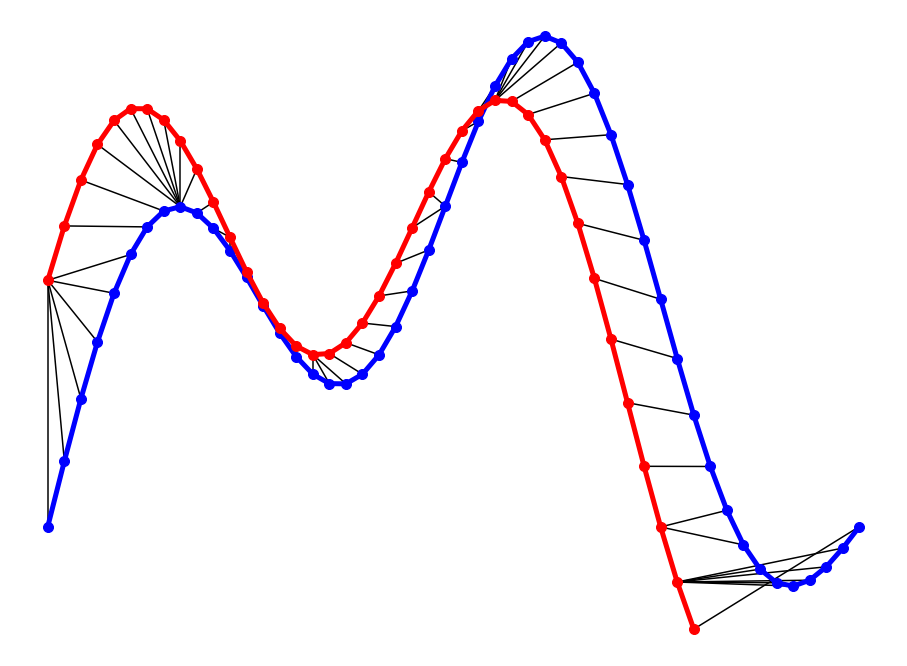In this post, we will go over the mathematics behind DTW. Then, two illustrative examples are provided to better understand the concept. If you are not interested in the math behind it, please jump to examples.

Dynamic Time Warping (DTW) is a way to compare two -usually temporal- sequences that do not sync up perfectly. It is a method to calculate the optimal matching between two sequences. DTW is useful in many domains such as speech recognition, data mining, financial markets, etc. It’s commonly used in data mining to measure the distance between two time-series.

In this post, we will go over the mathematics behind DTW. Then, two illustrative examples are provided to better understand the concept. If you are not interested in the math behind it, please jump to examples.

## Formulation

Let’s assume we have two sequences like the following:

𝑋=𝑥, 𝑥, …, x[i], …, x[n]

Y=y, y, …, y[j], …, y[m]

The sequences 𝑋 and 𝑌 can be arranged to form an 𝑛-by-𝑚 grid, where each point (𝑖, j) is the alignment between 𝑥[𝑖] and 𝑦[𝑗].

A warping path 𝑊 maps the elements of 𝑋 and 𝑌 to minimize the distance between them. 𝑊 is a sequence of grid points (𝑖, 𝑗). We will see an example of the warping path later.

### Warping Path and DTW distance

The Optimal path to (𝑖_𝑘, 𝑗_𝑘) can be computed by: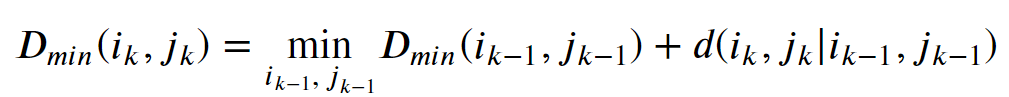where 𝑑 is the Euclidean distance. Then, the overall path cost can be calculated as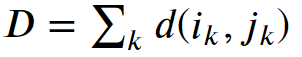## Restrictions on the Warping function

The warping path is found using a dynamic programming approach to align two sequences. Going through all possible paths is “combinatorically explosive” . Therefore, for efficiency purposes, it’s important to limit the number of possible warping paths, and hence the following constraints are outlined:

• Boundary Condition: This constraint ensures that the warping path begins with the start points of both signals and terminates with their endpoints.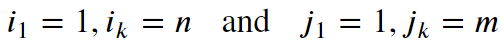• Monotonicity condition: This constraint preserves the time-order of points (not going back in time).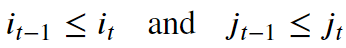• Continuity (step size) condition: This constraint limits the path transitions to adjacent points in time (not jumping in time).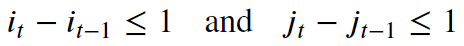In addition to the above three constraints, there are other less frequent conditions for an allowable warping path:

• Warping window condition: Allowable points can be restricted to fall within a given warping window of width 𝜔 (a positive integer).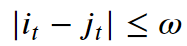• Slope condition: The warping path can be constrained by restricting the slope, and consequently avoiding extreme movements in one direction.

An acceptable warping path has combinations of chess king moves that are:

• Horizontal moves: (𝑖, 𝑗) → (𝑖, 𝑗+1)
• Vertical moves: (𝑖, 𝑗) → (𝑖+1, 𝑗)
• Diagonal moves: (𝑖, 𝑗) → (𝑖+1, 𝑗+1)

## Time Series Pattern Recognition with Air Quality Sensor Data

A real-world client-facing project with real sensor data.In the project, there are two data sets, each consists of one week of sensor readings are provided to accomplish the following four tasks.

## Applied Data Science with Python Certification Training Course -IgmGuru

Master Applied Data Science with Python and get noticed by the top Hiring Companies with IgmGuru's Data Science with Python Certification Program. Enroll Now

## Data Science : Time Series Analysis using Python

What is a Time Series? Depending on the frequency of observations, a time series may typically be hourly, daily, weekly, monthly, quarterly and annual. Sometimes, you might have seconds and minute-wise time series as well, like, number of clicks and user visits every minute etc.

## How I Built Time Series Data Out of Cross-Sectional Uber Travel Times Data

I knew I wanted to do two things in the process of writing my bachelor’s thesis: improve my programming skills and work with time-series data prediction.

## Preparing data for time series analysis

TS may look like a simple data object and easy to deal with, but the reality is that for someone new it can be a daunting task just to prepare the dataset before the actual fun stuff can begin.